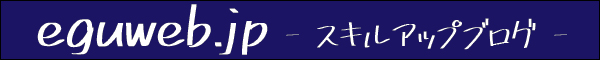# What is cost of sales? Calculation method and journal entry method

This time, I will introduce one of the finances, ” Cost of sales (Uriage Genka) “.

## What is cost of sales?

Cost of sales (Uriagegenka) is the cost of purchasing goods and services.

In the account item (bookkeeping 3rd grade), the following is the cost of sales.

• purchase amount
• purchase rebate

## How to find the cost of sales

The cost of goods sold can be calculated using the following method.

Cost of sales = Product inventory at the beginning of the period + Product purchases at the end of the period – Product inventory at the end

Term-end merchandise inventories are recorded as inventories at the end of the term, and are subtracted because they are not included in the cost of sales for the term.

## Cost of sales example

If 800 items were purchased at the beginning of the period at 100 yen each in the previous period, and 200 items were purchased at 100 yen each during the period, and 100 items remained in inventory at the end of the period.

Calculate by applying the above formula.

Cost of sales = Product inventory at the beginning of the period (800 units x 100 yen) + Product purchases for the current period (100 units x 100 yen) – Product inventory at the end of the period 100 units x 100 yen

For the sake of clarity, all purchase prices are the same amount.

Cost of sales = 80,000 + 20,000 – 10,000 = 90,000 yen

If the products excluding the products at the beginning of the term are sold for 500 yen each, the sales will be as follows.

Sales = 800 x 500 = ¥400,000

The profit will be:

Gross profit = JPY 400,000 (Sales) – JPY 90,000 (Current cost) = JPY 310,000